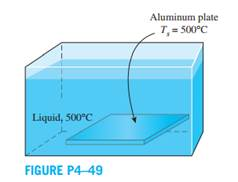Create an Account

Home / Questions / A 10cm thick aluminum plate

A 10cm thick aluminum plate

A 10-cm thick aluminum plate (ρ = 2702 kg/m3 , cp = 903 J/kg·K, k = 237 W/m·K, and α = 97.1 × 10–6 m2 /s) is being heated in liquid with temperature of 500°C. The aluminum plate has a uniform initial temperature of 25°C. If the surface temperature of the aluminum plate is approximately the liquid temperature, determine the temperature at the center plane of the aluminum plate after 15 seconds of heating. Solve this problem using analytical one-term approximation method (not the Heisler charts).Jul 24 2020 View more View LessSubscribe To Get Solution